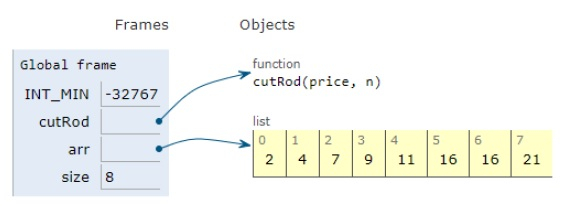# Python Program for Cutting a Rod

Problem statement − We are given a rod of length n and an array of prices that contains prices of all pieces of the size which are smaller than n. We need to determine the maximum value obtainable by cutting up the rod and selling its pieces.

We will be using a dynamic programming approach to solve the problem.

Now let’s observe the solution in the implementation below−

## Example

Live Demo

# A Dynamic Programming solution for Rod cutting problem
INT_MIN = -32767
# cut function
def cutRod(price, n):
val = [0 for x in range(n + 1)]
val = 0
# bottom up manner
for i in range(1, n + 1):
max_val = INT_MIN
for j in range(i):
max_val = max(max_val, price[j] + val[i-j-1])
val[i] = max_val
return val[n]
# main
arr = [2, 4, 7, 9, 11, 16, 16, 21]
size = len(arr)
print("Maximum Obtainable Value is " + str(cutRod(arr, size)))

## Output

Maximum Obtainable Value is 21All the variables are declared in the local scope and their references are seen in the figure above.

## Conclusion

In this article, we have learned about how we can make a Python Program for Cutting a Rod.